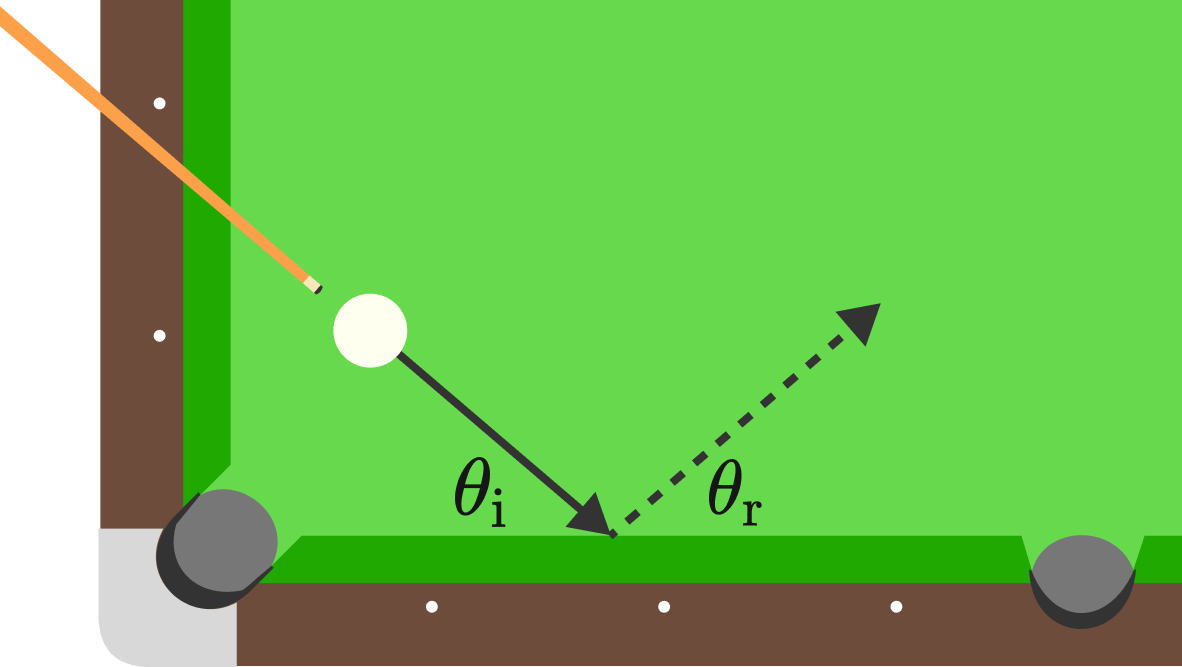# Cushion Shot in Billiards

Suppose we aim the cue ball at the cushion with some angle of incidence $\theta_\textrm{i} \neq 90^\circ.$ How will $\theta_\textrm{i}$ compare to $\theta_\textrm{r},$ the angle of reflection?

Naively it may seem that we can apply the law of reflection (that the angle of incidence is equal to the angle of reflection), but in practice we can't since some energy is lost upon colliding with the wall.Details and Assumptions:

• For simplicity, take the cue ball to be a point particle that has no sliding friction with the wall.
• The ball doesn't curve or jump on its way to the wall.
×